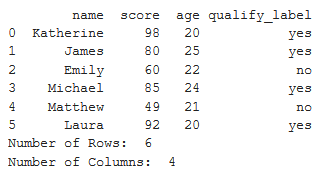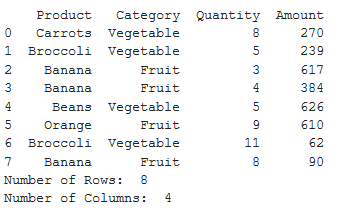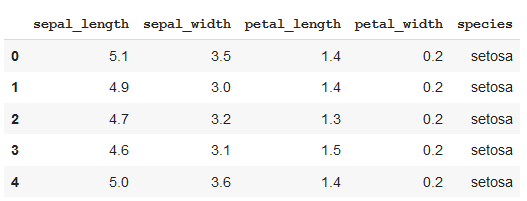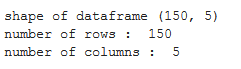# Count the number of rows and columns of a Pandas dataframe

Pandas allow us to get the shape of the dataframe by counting the numbers of rows and columns in the dataframe. You can try various approaches to get the number of rows and columns of the dataframe. All of them have been discussed below.

Example: Let’s take an example of a dataframe which consists of data of exam result of students.

 `# importing pandas  ` `import` `pandas as pd ` `result_data ``=` `{``'name'``: [``'Katherine'``, ``'James'``, ``'Emily'``, ` `                        ``'Michael'``, ``'Matthew'``, ``'Laura'``], ` `        ``'score'``: [``98``, ``80``, ``60``, ``85``, ``49``, ``92``], ` `        ``'age'``: [``20``, ``25``, ``22``, ``24``, ``21``, ``20``], ` `        ``'qualify_label'``: [``'yes'``, ``'yes'``, ``'no'``, ` `                          ``'yes'``, ``'no'``, ``'yes'``]} ` ` `  `# creating dataframe ` `df ``=` `pd.DataFrame(result_data, index ``=` `None``) ` ` `  `# computing number of rows ` `rows ``=` `len``(df.axes[``0``]) ` ` `  `# computing number of columns ` `cols ``=` `len``(df.axes[``1``]) ` ` `  `print``(df) ` `print``(``"Number of Rows: "``, rows) ` `print``(``"Number of Columns: "``, cols) `

Output :Example: Let’s take an example of a dataframe that consists of data on product sales.

 `# importing pandas  ` `import` `pandas as pd ` ` `  `# creating dataframe ` `df ``=` `pd.DataFrame({``'Product'` `: [``'Carrots'``, ``'Broccoli'``, ``'Banana'``,  ` `                                ``'Banana'``, ``'Beans'``, ``'Orange'``, ` `                                ``'Broccoli'``, ``'Banana'``], ` `                   ``'Category'` `: [``'Vegetable'``, ``'Vegetable'``, ``'Fruit'``,  ` `                                 ``'Fruit'``, ``'Vegetable'``, ``'Fruit'``,  ` `                                 ``'Vegetable'``, ``'Fruit'``], ` `                   ``'Quantity'` `: [``8``, ``5``, ``3``, ``4``, ``5``, ``9``, ``11``, ``8``], ` `                   ``'Amount'` `: [``270``, ``239``, ``617``, ``384``, ``626``, ``610``, ``62``, ``90``]}) ` ` `  `print``(df) ` ` `  `# getting number of rows ` `print``(``"Number of Rows: "``, ``len``(df.axes[``0``])) ` ` `  `# getting number of columns ` `print``(``"Number of Columns: "``, ``len``(df.axes[``1``])) `

Output :Example: Here, we will try a different approach for calculating rows and columns of a dataframe of imported csv file.

 `# importing pandas ` `import` `pandas as pd ` ` `  `# importing csv file ` `df ``=` `pd.read_csv(``'https://raw.githubusercontent.com/uiuc-cse/data-fa14/gh-pages/data/iris.csv'``) ` ` `  `print``(df.head()) ` ` `  `# obtaining the shape ` `print``(``"shape of dataframe"``, df.shape) ` ` `  `# obtaining the number of rows ` `print``(``"number of rows : "``, df.shape[``0``]) ` ` `  `# obtaining the number of columns ` `print``(``"number of columns : "``, df.shape[``1``]) `

Output :My Personal Notes arrow_drop_upCheck out this Author's contributed articles.

If you like GeeksforGeeks and would like to contribute, you can also write an article using contribute.geeksforgeeks.org or mail your article to contribute@geeksforgeeks.org. See your article appearing on the GeeksforGeeks main page and help other Geeks.

Please Improve this article if you find anything incorrect by clicking on the "Improve Article" button below.

Article Tags :

Be the First to upvote.

Please write to us at contribute@geeksforgeeks.org to report any issue with the above content.